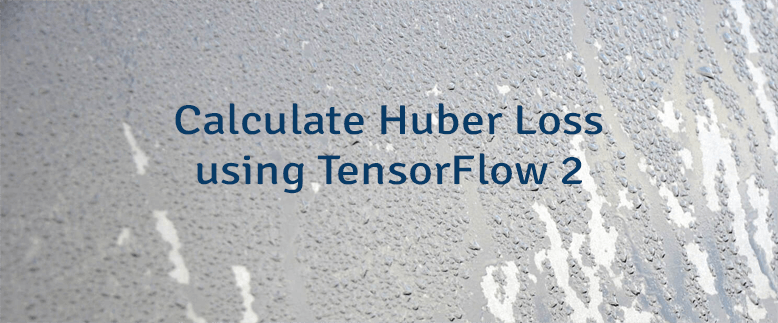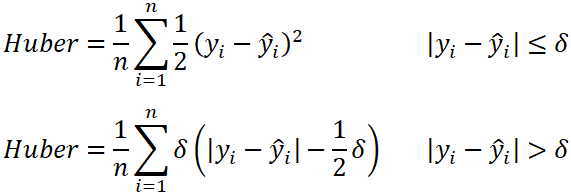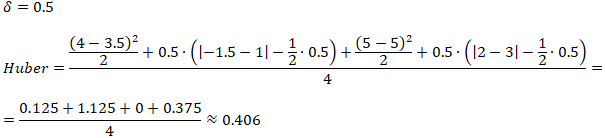# Calculate Huber Loss using TensorFlow 2

Huber loss is a loss function that is used to solve regression problems. This function is a combination of the mean squared error (MSE) and mean absolute error (MAE). Huber loss function is quadratic (MSE) when difference between actual and predicted values is small, otherwise function is linear (MAE). Parameter δ (delta) defines the point where the function transitions from a quadratic to linear.

The formula to calculate the Huber loss:• `n` - the number of data points.
• `y` - the actual value of the data point. Also known as true value.
• `ŷ` - the predicted value of the data point. This value is returned by model.
• `δ` - defines the point where the Huber loss function transitions from a quadratic to linear.

Let's say we have the following sets of numbers:

Here is example how Huber loss can be calculated using these numbers then δ is 0.5:TensorFlow 2 allows to calculate the Huber loss. It can be done by using `Huber` class.

``````from tensorflow import keras

yActual = [4, -1.5, 5, 2]
yPredicted = [3.5, 1, 5, 3]

huberObject = keras.losses.Huber(delta=0.5)
huberTensor = huberObject(yActual, yPredicted)
huber = huberTensor.numpy()

print(huber)``````

Huber loss also can be calculated by using `huber` function.

``````huberTensor = keras.losses.huber(yActual, yPredicted, delta=0.5)
huber = huberTensor.numpy()``````